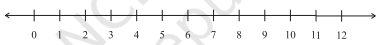# Between which two whole numbers on the number line are the given numbers lie? Which of these whole numbers is nearer the number?(a) 0.8 (b) 5.1 (c) 2.6 (d) 6.4 (e) 9.1 (f) 4.9"

To do:

We have to find between which two whole numbers on the number line the given numbers lie.

Solution:

(a) $0<0.8<1$

This implies,

$0.8$ lies between 0 and 1.

0.8 is nearer to 1.

(b) $5<5.1<6$

This implies,

$5.1$ lies between the whole numbers 5 and 6.

5.1 is nearer to 5.

(c) $2<2.6<3$

This implies,

$2.6$ lies between the whole numbers 2 and 3.

2.6 is nearer to 3.

(d) $6<6.4<7$

This implies,

$6.4$ lies between the whole numbers 6 and 7.

6.4 is nearer to 6.

(e) $9<9.1<10$

This implies,

$9.1$ lies between the whole numbers 9 and 10.

9.1 is nearer to 9.

(f) $4<4.9<5$

This implies,

$4.9$ lies between the whole numbers 4 and 5.

4.9 is nearer to 5.